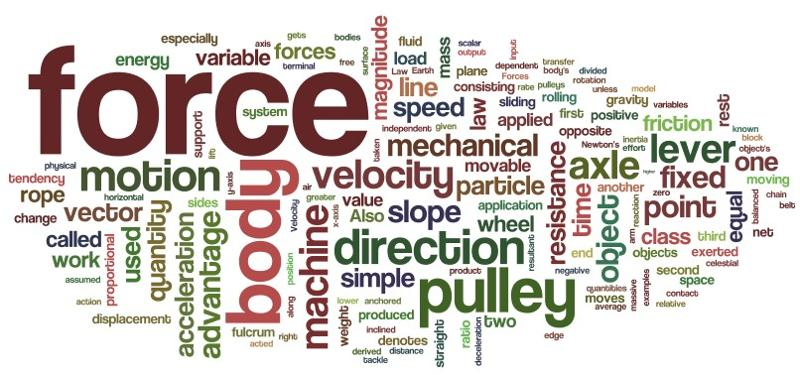Engineering Entrance Sample Papers

Conservation of Linear Momentum Questions and AnswersLinear Momentum: Conservation of Momentum Physics Multiple Choice Questions:

Ques. A body at rest breaks into two pieces of equal masses. The parts will move
(a) in same direction
(b) along different lines
(c) in opposite directions with equal speeds
(d) in opposite directions with unequal speeds.
Ans. (c)

Related: Surface chemistry MCQ

Ques. Consider the following two statements:
A) Linear momentum of the system remains constant.
B) Centre of mass of the system remains at rest.
(a) A implies B and B implies A.
(b) A does not imply B and B does not imply A.
(c) A implies B but B does not imply A.
(d) B implies A but A does not imply B.
Ans. (d)

Ques. Two bodies are projected from roof with same speed in different directions. If air resistance is not taken into account then:
(a) they reach at ground with same magnitude of momenta if bodies have same masses
(b) they reach at ground with same kinetic energy
(c) they reach at ground with same speed
(d) both (a) and (c) correct
Ans. (d)

Ques. The hero of a stunt film fires 50 g bullets from a machine gun, each at a speed of 1.0 km/s. If he fires 20 bullets in 4 seconds, what average force does he exert against the machine gun during this period ?
(a) 200 N
(b) 220 N
(c) 250 N
(d) 270 N
Ans. (c)

Ques. A mass m with velocity u strikes a wall normally and returns with the same speed. What is magnitude of the change in momentum of the body when it returns?
(a) 2mu
(b) 3mu
(c) 4mu
(d) 5mu
Ans. (a)

Ques. A boy of mass 25 kg stands on a board of mass 10 kg which in turn is kept on a frictionless horizontal ice surface. The boy makes a jump with a velocity component 5 m/s in a horizontal direction with respect to the ice. With what velocity does the board recoil ? With what rate are the boy and the board separating from each other?
(a) 12.5 m/s
(b) 27.5 m/s
(c) 18.5 m/s
(d) 17.5 m/s
Ans. (d)

Ques. A projectile is fired from a gun at an angle of 45° with the horizontal and with a speed of 20 m/s relative to ground. At the highest point in its flight the projectile explodes into two fragments of equal mass. One fragment, whose initial speed is zero falls vertically. How far from the gun does the other fragment land, assuming a level terrain ? [Take g = 10 m/s2 ]
(a) 60 m
(b) 65 m
(c) 55 m
(d) 70 m
Ans. (a)

Ques. A boy of mass 60 kg is standing over a platform of mass 40 kg placed over a smooth horizontal surface. He throws a stone of mass 1 kg with velocity v = 10 m/s at an angle of 45° with respect to the ground. Find the displacement of the platform (with boy) on the horizontal surface when the stone lands on the ground. [g = 10 m/s2].
(a) 18 cm
(b) 10 cm
(c) 12 cm
(d) 15 cm
Ans. (b)

Ques. A shell is fired from a cannon with a velocity of 50 ms–1 at an angle 60° to the horizontal. At the highest point of its path it splits up into three equal parts; one part retraces its path; the other falls down vertically. What is the velocity of the third part ?
(a) 200 ms–1
(b) 150 ms–1
(c) 100 ms–1
(d) 50 ms–1
Ans. (c)

Related: MCQ on Animal tissues

Ques. A space shuttle, while travelling at a speed of 4000 km/h with respect to the earth, disconnects and ejects a module backward, weighing one fifth of the residual part. If the shuttle ejects the disconnected module at a speed 100 km/h with respect to the state of the shuttle before the ejection, find the final velocity of the shuttle.
(a) 4000 km/h
(b) 4010 km/h
(c) 4020 km/h
(d) 4030 km/h
Ans. (c)

Ques. The momentum of a boy of 60 Kg weight running at 10 m/s is
(a) 500 Kg m/s
(b) 600 Kg m/s
(c) 550 Kg m/s
(d) 450 Kg m/s
Ans. (b)